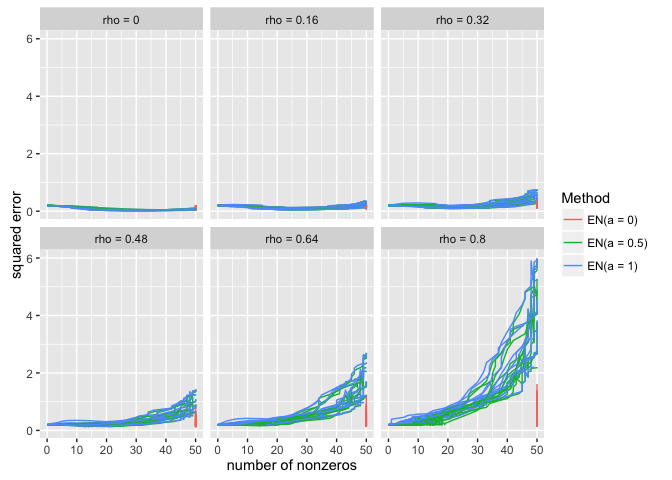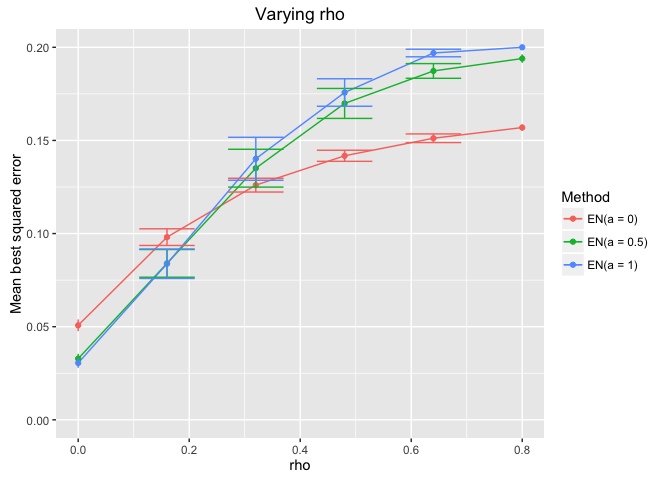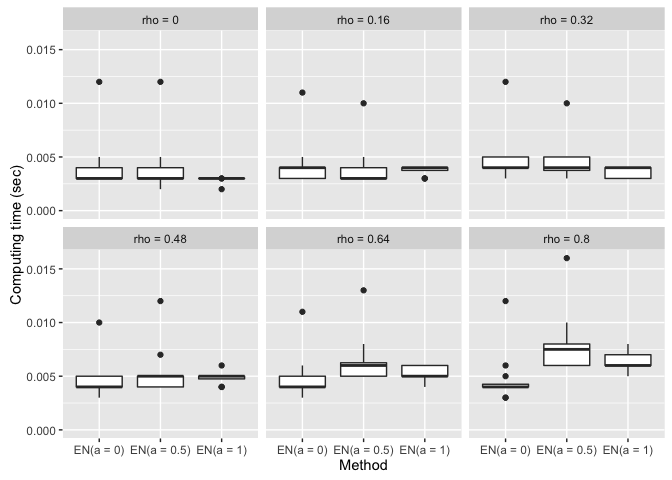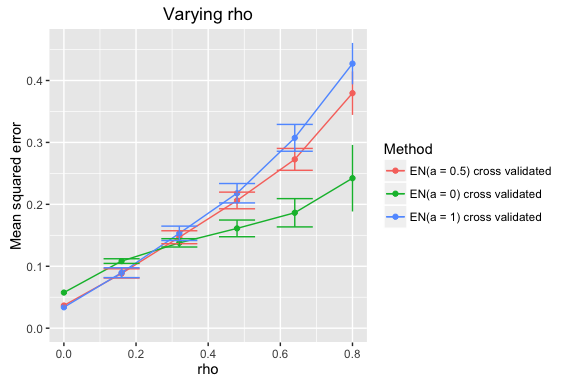# The Elastic Net with the simulator

#### 2016-07-12

In this vignette, we perform a simulation with the elastic net to demonstrate the use of the simulator in the case where one is interested in a sequence of methods that are identical except for a parameter that varies. The elastic net is the solution $$\hat\beta_{\lambda,\alpha}$$ to the following convex optimization problem: $\min_{\beta\in\mathbb R^p}\frac1{2}\|y-X\beta\|_2^2+\lambda(1-\alpha)\|\beta\|^2_2+\lambda\alpha\|\beta\|_1.$

Here, $$\lambda\ge0$$ controls the overall amount of regularization whereas $$\alpha\in[0,1]$$ controls the tradeoff between the lasso and ridge penalties. While sometimes one performs a two-dimensional cross-validation over $$(\lambda,\alpha)$$ pairs, in some simulations one might wish instead to view each fixed $$\alpha$$ as corresponding to a separate version of the elastic net (each solved along a grid of $$\lambda$$ values). Such a view is useful for understanding the effect $$\alpha$$.

# Main simulation

## Understanding the effect of the elastic net’s $$\alpha$$ parameter

We begin with a simulation showing the best-case performance of the elastic net for several values of $$\alpha$$.

library(simulator)
name_of_simulation <- "elastic-net"
sim <- new_simulation(name_of_simulation, "Elastic Nets") %>%
generate_model(make_sparse_linear_model_with_corr_design,
n = 100, p = 50, snr = 2, k = 10,
rho = as.list(seq(0, 0.8, length = 6)),
vary_along = "rho") %>%
simulate_from_model(nsim = 3, index = 1:4) %>%
run_method(list_of_elastic_nets,
parallel = list(socket_names = 2, libraries = "glmnet")) %>%
evaluate(list(sqr_err, nnz, best_sqr_err))

In the above code, we consider a sequence of models in which we vary the correlation rho among the features. For each model, we fit a sequence of elastic net methods (varying the tuning parameter $$\alpha$$). For each method, we compute the best-case mean-squared error. By best-case, we mean $$\min_{\lambda\ge0}\frac1{p}\|\hat\beta_{\lambda,\alpha}-\beta\|_2^2$$, which imagines we have an oracle-like ability to choose the best $$\lambda$$ for minimizing the MSE.

We provide below all the code for the problem-specific components. We use the R package glmnet to fit the elastic net. The most distinctive feature of this particular vignette is how the list of methods list_of_elastic_nets was created. This is shown in the Methods section.

plot_evals(sim, "nnz", "sqr_err")The first plot shows the MSE versus sparsity level for each method (parameterized by $$\lambda$$). As expected, we see that when $$\alpha=1$$ (pure ridge regression), there is no sparsity. We see that the performance of the methods with $$\alpha<1$$ degrades as the correlation among features increases, especially when a lot of features are included in the fitted model.

It is informative to look at how the height of the minimum of each of the above curves varies with $$\rho$$.

plot_eval_by(sim, "best_sqr_err", varying = "rho", include_zero = TRUE)We see that when the correlation between features is low, the methods with some $$\ell_1$$ penalty do better than ridge regression. However, as the features become increasingly correlated, a pure ridge penalty becomes better. Of course, none of the methods are doing as well in the high correlation regime (which is reminiscent of the bet on sparsity principle).

A side note: the simulator automatically records the computing time of each method as an additional metric:

plot_eval(sim, "time", include_zero = TRUE)## Results for Cross-Validated Elastic Net

We might be reluctant to draw conclusions about the methods based on the oracle-like version that we used above (in which each method on each random draw gets to pick the best possible $$\lambda$$ value). We might therefore look at the performance of the methods using cross-validation to select $$\lambda$$.

sim_cv <- sim %>% subset_simulation(methods = "") %>%
rename("elastic-net-cv") %>%
relabel("Elastic Nets with CV") %>%
run_method(list_of_elastic_nets + cv,
parallel = list(socket_names = 2, libraries = "glmnet")) %>%
evaluate(list(sqr_err, nnz))

Reassuringly, the relative performance of these methods is largely the same (though we see that all methods’ MSEs are higher).

plot_eval_by(sim_cv, "sqr_err", varying = "rho", include_zero = TRUE)# Components

The most distinctive component in this vignette is in the Methods section. Rather than directly creating a Method object, we write a function that creates a Method object. This allows us to easily create a sequence of elastic net methods that differ only in their setting of the $$\alpha$$ parameter.

## Models

library(mvtnorm)
make_sparse_linear_model_with_corr_design <- function(n, p, k, snr, rho) {
sig <- matrix(rho, p, p)
diag(sig) <- 1
x <- rmvnorm(n, sigma = sig)
beta <- rep(c(1, 0), c(k, p - k))
mu <- as.numeric(x %*% beta)
sigma <- sqrt(sum(mu^2) / (n * snr)) # taking snr = ||mu||^2 / (n * sigma^2)
new_model(name = "slm", label = sprintf("rho = %s", rho),
params = list(x = x, beta = beta, mu = mu, sigma = sigma, n = n,
p = p, k = k),
simulate = function(mu, sigma, nsim) {
y <- mu + sigma * matrix(rnorm(nsim * n), n, nsim)
return(split(y, col(y))) # make each col its own list element
})
}

## Methods

library(glmnet)
make_elastic_net <- function(alpha) {
new_method(name = sprintf("en%s", alpha),
label = sprintf("EN(a = %s)", alpha),
settings = list(alpha = alpha, nlam = 50),
method = function(model, draw, alpha, nlam, lambda = NULL) {
if (is.null(lambda))
fit <- glmnet(x = model$x, y = draw, alpha = alpha, nlambda = nlam, intercept = FALSE) else fit <- glmnet(x = model$x, y = draw, alpha = alpha,
lambda = lambda, intercept = FALSE)
list(beta = fit$beta, yhat = model$x %*% fit$beta, lambda = fit$lambda, df = fit$df, alpha = alpha) }) } list_of_elastic_nets <- sapply(c(0, 0.5, 1), make_elastic_net) The function make_elastic_net takes a value of $$\alpha$$ and creates a Method object corresponding to the elastic net with that value of $$\alpha$$. In the second set of simulations, we studied cross-validated versions of each elastic net method. To do this, we wrote list_of_elastic_nets + cv. This required writing the following MethodExtension object cv. The vignette on the lasso has more about writing method extensions. #' Make folds for cross validation #' #' Divides the indices \code{1:n} into \code{nfolds} random folds of about the same size. #' #' @param n sample size #' @param nfolds number of folds make_folds <- function(n, nfolds) { nn <- round(n / nfolds) sizes <- rep(nn, nfolds) sizes[nfolds] <- sizes[nfolds] + n - nn * nfolds b <- c(0, cumsum(sizes)) ii <- sample(n) folds <- list() for (i in seq(nfolds)) folds[[i]] <- ii[seq(b[i] + 1, b[i + 1])] folds } cv <- new_method_extension("cv", "cross validated", method_extension = function(model, draw, out, base_method) { nfolds <- 5 alpha <- base_method@settings$alpha
err <- matrix(NA, ncol(out$beta), nfolds) ii <- make_folds(model$n, nfolds)
for (i in seq_along(ii)) {
train <- model
train@params$x <- model@params$x[-ii[[i]], ]
train@params$n <- model@params$x[-ii[[i]], ]
train_draw <- draw[-ii[[i]]]

test <- model
test@params$x <- model@params$x[ii[[i]], ]
test@params$n <- model@params$x[ii[[i]], ]
test_draw <- draw[ii[[i]]]
fit <- base_method@method(model = train,
draw = train_draw,
alpha = alpha,
lambda = out$lambda) yhat <- test$x %*% fit$beta ll <- seq(ncol(yhat)) err[ll, i] <- colMeans((yhat - test_draw)^2) } m <- rowMeans(err) se <- apply(err, 1, sd) / sqrt(nfolds) imin <- which.min(m) ioneserule <- max(which(m <= m[imin] + se[imin])) list(err = err, m = m, se = se, imin = imin, ioneserule = ioneserule, beta = out$beta[, imin],
yhat = model$x %*% out$beta[, imin],
alpha = alpha)
})

## Metrics

sqr_err <- new_metric("sqr_err", "squared error",
metric = function(model, out) {
colMeans(as.matrix(out$beta - model$beta)^2)
})

best_sqr_err <- new_metric("best_sqr_err", "best squared error",
metric = function(model, out) {
min(colMeans(as.matrix(out$beta - model$beta)^2))
})

nnz <- new_metric("nnz", "number of nonzeros",
metric = function(model, out) {
colSums(as.matrix(out\$beta) != 0)
})

# Conclusion

To cite the simulator, please use

citation("simulator")

Bien J (2016). “The simulator: An Engine to Streamline Simulations.” Submitted. <URL: http://faculty.bscb.cornell.edu/~bien/simulator.pdf>.

A BibTeX entry for LaTeX users is

@Article{, title = {The {simulator}: An Engine to Streamline Simulations}, author = {Jacob Bien}, year = {2016}, url = {http://faculty.bscb.cornell.edu/~bien/simulator.pdf}, journal = {Submitted}, }